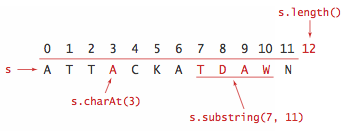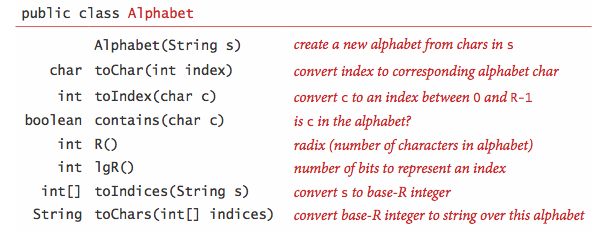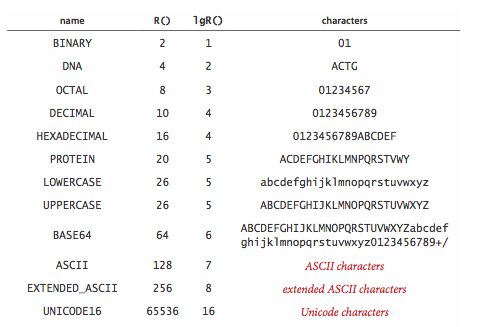# 5.   Strings

## Overview.

We communicate by exchanging strings of characters. We consider classic algorithms for addressing the underlying computational challenges surrounding applications such as the following:
• 5.1 String Sorts includes LSD radix sort, MSD radix sort, and 3-way radix quicksort for sorting arrays of strings.
• 5.2 Tries describes R-way tries and ternary search tries for implementing symbol tables with string keys.
• 5.3 Substring Search describes algorithms for searching for a substring in a large piece of text, including the classic Knuth-Morris-Pratt, Boyer-Moore, and Rabin-Karp algorithms.
• 5.4 Regular Expressions introduces a quintessential search tool known as grep that we use to search for incompletely specified substrings.
• 5.5 Data Compression introduces data compression, where we try to reduce the size of a string to the minimum possible. We present the classic Huffman and LZW algorithms.

## Rules of the game.

For clarity and efficiency, our implementations are expressed in terms of the Java String class. We briefly review their most important characteristics.

• Characters. A String is a sequence of characters. Characters are of type char and can have one of 2^16 possible values. For many decades, programmers restricted attention to characters encoded in 7-bit ASCII or 8-bit extended ASCII, but many modern applications call for 16-bit Unicode.

• Immutability. String objects are immutable, so that we can use them in assignment statements and as arguments and return values from methods without having to worry about their values changing.

• Indexing. The charAt() method extracts a specified character from a string in constant time.

• Length. The length() method returns the length of a string in constant time.

• Substring. The substring() method typically extracts a specified substring in constant time.

WARNING: Beginning with Oracle and OpenJDK Java 7, Update 6, the substring() method takes linear time and space in the size of the extracted substring. Since we did not anticipate this drastic change, some of our string-processing code will suffer the consequences. The String API provides no performance guarantees for any of its methods, including substring() and charAt(). The lesson is to use at your own risk.• Concatenation. The + operator performs string concatenation. We avoid forming a string by appending one character at a time because that is a quadratic-time process in Java. (Java has a StringBuilder class for that use.)

• Character arrays. The Java String is not a primitive type. The standard implementation provides the operations just described to facilitate client programming. By contrast, many of the algorithms that we consider can work with a low-level representation such as an array of char values, and many clients might prefer such a representation, because it consumes less space and takes less time.

## Alphabets.

Some applications involve strings taken from a restricted alphabet. In such applications, it often makes sense to use an Alphabet.java class with the following API:The constructor that takes as argument an R-character string that specifies the alphabet; the toChar() and toIndex() methods convert (in constant time) between string characters and int values between 0 and R-1. The method R() returns the number of characters in the alphabet or radix. A number of predefined alphabets are included:Count.java is a client that takes an alphabet specified on the command line, reads in a sequence of characters over that alphabet (ignoring characters not in the alphabet), computes the frequency of occurrence of each character,

## Java programs in this chapter.

Below is a list of Java programs in this chapter. Click on the program name to access the Java code; click on the reference number for a brief description; read the textbook for a full discussion.

- Alphabet.java alphabet
- Count.java alphabet client
- InplaceMSD.java In-place MSD radix sort1
5.3 Quick3string.java 3-way string quicksort
- AmericanFlag.java American flag sort1
- AmericanFlagX.java non-recursive American flag sort1
5.4 TrieST.java multiway trie symbol table
- TrieSET.java multiway trie set
5.5 TST.java ternary search trie
5.6 KMP.java substring search (Knuth–Morris–Pratt)
5.7 BoyerMoore.java substring search (Boyer–Moore)
5.8 RabinKarp.java substring search (Rabin–Karp)
5.9 NFA.java NFA for regular expressions
- GREP.java grep
- BinaryDump.java binary dump
- HexDump.java hex dump
- PictureDump.java picture dump
- Genome.java genomic code
- RunLength.java data compression (run-length coding)
5.10 Huffman.java data compression (Huffman)
5.11 LZW.java data compression (Lempel–Ziv–Welch)

#### Q + A

Q.What is Unicode.

A. Unicode (Universal character encoding) = complex 21-bit code to represent International symbols and other characters.

Q.What is UTF-16.

A. UTF-16 (Unicode Transformation Format) = complex 16-bit variable width code to represent Unicode characters. Most common characters are represented using 16 bits (a char), but surrogate pairs are represented using a pair of char values. If first char value is between D800 and DFFF, then it is combined with the next char (in the same range) to form a surrogate pair. No Unicode characters correspond to D800 through DFFF. For example, 007A represents a lowercase Z, 6C34 represents the Chinese symbol for water, and D834 DD1E represents the musical G clef.

Q. What's the substring trap?

A. The String method call s.substring(i, j) returns the substring of s starting at index i and ending at j-1 (not at j as you might suspect).

Q. How can I change the value of a string?

A. You can't since strings are immutable in Java. If you want a new string, then you must create a new one using string concatenation or one of the string methods that returns a new string such as toLowerCase() or substring().

#### Web Exercises

1. Squeeze whitespace. Write a program Squeeze.java that takes as input a string and removes adjacent spaces, leaving at most one space in-a-row.
2. Remove duplicates. Given a string, create a new string with all the consecutive duplicates removed. For example, ABBCCCCCBBAB becomes ABCBAB.
3. String of N x's. Describe the string that the following function returns, given a positive integer N?
 public static String mystery(int N) { String s = ""; while(N > 0) { if (N % 2 == 1) s = s + s + "x"; else s = s + s; N = N / 2; } return s; } 
4. Palindrome check. Write a function that takes as input a string and returns true if the string is a palindrome, and false otherwise. A palindrome is a string that reads the same forwards or backwards.
5. Watson-Crick complemented palindrome check. Write a function that takes as input a string and returns true if the string is a Watson-Crick complemented palindrome, and false otherwise. A Watson-Crick complemented palindrome is a DNA string that is equal to the complement (A-T, C-G) of its reverse.
6. Watson-Crick complement. Write a function that takes as input a DNA string of A, C, G, and T characters and returns the string in reverse order with all of characters replaced by their complements. For example, if the input is ACGGAT, then return ATCCGT.
7. Perfect shuffle. What does the following recursive function return, given two strings s and t of the same length?
 public static String mystery(String s, String t) { int N = s.length(); if (N <= 1) return s + t; String a = mystery(s.substring(0, N/2), t.substring(0, N/2)); String b = mystery(s.substring(N/2, N), t.substring(N/2, N)); return a + b; } 
8. Binary tree representation. Write a data type TreeString.java that represents an immutable string using a binary tree. It should support concatenation in constant time, and printing out the string in time proportional to the number of characters.
9. Reverse a string. Write a recursive function to reverse a string. Do not use any loops. Hint: use the String method substring().
 public static String reverse(String s) { int N = s.length(); if (N <= 1) return s; String a = s.substring(0, N/2); String b = s.substring(N/2, N); return reverse(b) + reverse(a); } 
How efficient is your method? Our method has a linearithmic running time.
10. Random string. Write a recursive function to create a random string of characters between 'A' and 'Z'.
 public static String random(int N) { if (N == 0) return ""; if (N == 1) return 'A' + StdRandom.uniform(26); return random(N/2) + random(N - N/2); } 
11. Subsequence. Given two strings s and t, write a program Subsequence.java that determines whether s is a subsequence of t. That is, the letters of s should appear in the same order in t, but not necessarily contiguously. For example accag is a subsequence of taagcccaaccgg.
12. Longest complemented palindrome. In DNA sequence analysis, a complemented palindrome is a string equal to its reverse complement. Adenine (A) and Thymine (T) are complements, as are Cytosine (C) and Guanine (G). For example, ACGGT is a complement palindrome. Such sequences act as transcription-binding sites and are associated with gene amplification and genetic instability. Given a text input of N characters, find the longest complemented palindrome that is a substring of the text. For example, if the text is GACACGGTTTTA then the longest complemented palindrome is ACGGT. Hint: consider each letter as the center of a possible palindrome of odd length, then consider each pair of letters as the center of a possible palindrome of even length.
13. DNA to RNA. Write a function that takes a DNA string (A, C, G, T) and returns the corresponding RNA string (A, C, G, U).
14. DNA complement. Write a function that takes as input a DNA string (A, C, G, T) and returns the complementary base pairs (T, G, C, A). DNA is typically found in a double helix structure. The two complementary DNA strands are joined in a spiral structure.

15. Convert from hexadecimal to decimal. Hex2Decimal.java contains a function takes a hexadecimal string (using A-F for the digits 11-15) and returns the corresponding decimal integer. It uses a number of the string library methods and Horner's method.
 public static int hex2decimal(String s) { String digits = "0123456789ABCDEF"; s = s.toUpperCase(); int val = 0; for (int i = 0; i < s.length(); i++) { char c = s.charAt(i); int d = digits.indexOf(c); val = 16*val + d; } return val; } 

Alternate solution: Integer.parseInt(String s, int radix). More robust, and works with negative integers.